latin square

Hall-Paige conjecture ★★★

Author(s): Hall; Paige

A complete map for a (multiplicative) groupis a bijection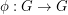so that the mapis also a bijection.

Conjecture   Ifis a finite group and the Sylow 2-subgroups ofare either trivial or non-cyclic, thenhas a complete map.

Keywords: complete map; finite group; latin square

Snevily's conjecture ★★★

Author(s): Snevily

Conjecture   Letbe an abelian group of odd order and let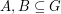satisfy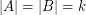. Then the elements ofandmay be orderedand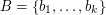so that the sums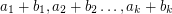are pairwise distinct.

Keywords: addition table; latin square; transversal

Even vs. odd latin squares ★★★

Author(s): Alon; Tarsi

A latin square is even if the product of the signs of all of the row and column permutations is 1 and is odd otherwise.

Conjecture   For every positive even integer, the number of even latin squares of orderand the number of odd latin squares of orderare different.

Keywords: latin square

Rota's basis conjecture ★★★

Author(s): Rota

Conjecture   Let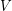be a vector space of dimensionand let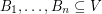be bases. Then there existdisjoint transversals ofeach of which is a base.

Keywords: base; latin square; linear algebra; matroid; transversal